x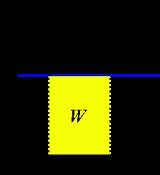Isobaric processOverview

An isobaric process is a thermodynamic process in which the pressure stays constant. The term derives from the Greek isos, (equal), and barus, (heavy). The heat transferred to the system does work but also changes the internal energy of the system: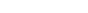According to the first law of thermodynamics
First law of thermodynamics
The first law of thermodynamics is an expression of the principle of conservation of work.The law states that energy can be transformed, i.e. changed from one form to another, but cannot be created nor destroyed...

, where W is work done by the system, U is internal energy, and Q is heat.Unanswered QuestionsWhat is the relevance of isobaric and isochoric process on engine operations?Encyclopedia
An isobaric process is a thermodynamic process in which the pressure stays constant. The term derives from the Greek isos, (equal), and barus, (heavy). The heat transferred to the system does work but also changes the internal energy of the system: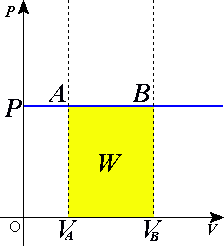According to the first law of thermodynamics
First law of thermodynamics
The first law of thermodynamics is an expression of the principle of conservation of work.The law states that energy can be transformed, i.e. changed from one form to another, but cannot be created nor destroyed...

, where W is work done by the system, U is internal energy, and Q is heat. Pressure-volume work by the closed system is defined as: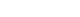where Δ means change over the whole process, whereas d denotes a differential. Since pressure is constant, this means that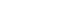.

Applying the ideal gas law
Ideal gas law
The ideal gas law is the equation of state of a hypothetical ideal gas. It is a good approximation to the behavior of many gases under many conditions, although it has several limitations. It was first stated by Émile Clapeyron in 1834 as a combination of Boyle's law and Charles's law...

, this becomes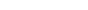assuming that the quantity of gas stays constant, e.g., there is no phase change during a chemical reaction
Chemical reaction
A chemical reaction is a process that leads to the transformation of one set of chemical substances to another. Chemical reactions can be either spontaneous, requiring no input of energy, or non-spontaneous, typically following the input of some type of energy, such as heat, light or electricity...

. According to the equipartition theorem
Equipartition theorem
In classical statistical mechanics, the equipartition theorem is a general formula that relates the temperature of a system with its average energies. The equipartition theorem is also known as the law of equipartition, equipartition of energy, or simply equipartition...

, the change in internal energy is related to the temperature of the system by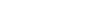,

where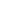is specific heat at a constant volume.

Substituting the last two equations into the first equation produces: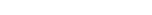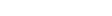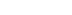,

where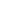is specific heat at a constant pressure.

## Specific heat capacity

To find the molar specific heat capacity of the gas involved, the following equations apply for any general gas that is calorically perfect. The property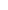is either called the adiabatic index or the heat capacity ratio
Heat capacity ratio
The heat capacity ratio or adiabatic index or ratio of specific heats, is the ratio of the heat capacity at constant pressure to heat capacity at constant volume . It is sometimes also known as the isentropic expansion factor and is denoted by \gamma or \kappa . The latter symbol kappa is...

. Some published sources might use k instead of.

Molar isochoric specific heat: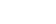.

Molar isobaric specific heat: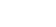.
The values forare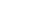for diatomic gasses like air and its major components, and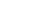for monatomic gasses like the noble gas
Noble gas
The noble gases are a group of chemical elements with very similar properties: under standard conditions, they are all odorless, colorless, monatomic gases, with very low chemical reactivity...

ses. The formulas for specific heats would reduce in these special cases:

Monatomic: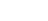and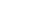Diatomic: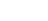and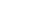An isobaric process is shown on a P-V diagram as a straight horizontal line, connecting the initial and final thermostatic states. If the process moves towards the right, then it is an expansion. If the process moves towards the left, then it is a compression.

## Sign convention for work

The motivation for the specific sign convention
Sign convention
In physics, a sign convention is a choice of the physical significance of signs for a set of quantities, in a case where the choice of sign is arbitrary. "Arbitrary" here means that the same physical system can be correctly described using different choices for the signs, as long as one set of...

s of thermodynamics
Thermodynamics
Thermodynamics is a physical science that studies the effects on material bodies, and on radiation in regions of space, of transfer of heat and of work done on or by the bodies or radiation...

comes from early development of heat engines. When designing a heat engine, the goal is to have the system produce and deliver work output. The source of energy in a heat engine, is a heat input.

If the volume compresses (delta V = final volume - initial volume < 0), then W < 0. That is, during isobaric compression the gas does negative work, or the environment does positive work. Restated, the environment does positive work on the gas.

If the volume expands (delta V = final volume - initial volume > 0), then W > 0. That is, during isobaric expansion the gas does positive work, or equivalently, the environment does negative work. Restated, the gas does positive work on the environment.

If heat is added to the system, then Q > 0. That is, during isobaric expansion/heating, positive heat is added to the gas, or equivalently, the environment receives negative heat. Restated, the gas receives positive heat from the environment.

If the system rejects heat, then Q < 0. That is, during isobaric compression/cooling, negative heat is added to the gas, or equivalently, the environment receives positive heat. Restated, the environment receives positive heat from the gas.

## Defining enthalpy

An isochoric process
Isochoric process
An isochoric process, also called a constant-volume process, an isovolumetric process, or an isometric process, is a thermodynamic process during which the volume of the closed system undergoing such a process remains constant...

is described by the equation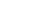. It would be convenient to have a similar equation for isobaric processes. Substituting the second equation into the first yields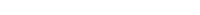The quantity U + p V is a state function so that it can be given a name. It is called enthalpy
Enthalpy
Enthalpy is a measure of the total energy of a thermodynamic system. It includes the internal energy, which is the energy required to create a system, and the amount of energy required to make room for it by displacing its environment and establishing its volume and pressure.Enthalpy is a...

, and is denoted as H. Therefore an isobaric process can be more succinctly described as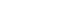.

Enthalpy and isobaric specific heat capacity are very useful mathematical constructs, since when analyzing a process in an open system, the situation of zero work occurs when the fluid flows at constant pressure. In an open system, enthalpy is the quantity which is useful to use to keep track of energy content of the fluid.

## Variable density viewpoint

A given quantity (mass m) of gas in a changing volume produces a change in density ρ. In this context the ideal gas law is written
R(T ρ) = M P

where T is thermodynamic temperature
Thermodynamic temperature
Thermodynamic temperature is the absolute measure of temperature and is one of the principal parameters of thermodynamics. Thermodynamic temperature is an "absolute" scale because it is the measure of the fundamental property underlying temperature: its null or zero point, absolute zero, is the...

. When R and M are taken as constant, then pressure P can stay constant as the density-temperature quadrant (ρ,T ) undergoes a squeeze mapping
Squeeze mapping
In linear algebra, a squeeze mapping is a type of linear map that preserves Euclidean area of regions in the Cartesian plane, but is not a Euclidean motion.For a fixed positive real number r, the mapping →...

.

## See also

• Adiabatic process
Adiabatic process
In thermodynamics, an adiabatic process or an isocaloric process is a thermodynamic process in which the net heat transfer to or from the working fluid is zero. Such a process can occur if the container of the system has thermally-insulated walls or the process happens in an extremely short time,...

• Cyclic process
• Isochoric process
Isochoric process
An isochoric process, also called a constant-volume process, an isovolumetric process, or an isometric process, is a thermodynamic process during which the volume of the closed system undergoing such a process remains constant...

• Isothermal process
Isothermal process
An isothermal process is a change of a system, in which the temperature remains constant: ΔT = 0. This typically occurs when a system is in contact with an outside thermal reservoir , and the change occurs slowly enough to allow the system to continually adjust to the temperature of the reservoir...

• Polytropic process
• Isoenthalpic process
The source of this article is wikipedia, the free encyclopedia.  The text of this article is licensed under the GFDL.